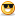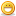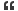# Thread: need Boundary Value analysis explaination with example

1. ##need Boundary Value analysis explaination with example

Hi,

How do you do BVA for range between 10-20?

This question was posed to me by a inteviewer. As per theory, i told
him 9,10,11 (min-1,min,min+ 1)and 19,20,21(max- 1,max,max+ 1)
values will be tested.Then he questioned the rationale behind testing
11 and 19 .he told me that if 11 passes surely 19 too would pass.for
which i was unable to reply.

Friendz,can anyone explain BVA clearly?????
Any docs on this subject would be very helpful.

Thanx,
vbReply With Quote

2. ## Re: need Boundary Value analysis explaination with example

Your initial answer was correct. He tried to confuse you by saying that if 11 passes then 19 will also pass. It is not necessarily true.

The logic behind BVA is that, for the reasons unknown, it is generally observed that the values near the boundaries (10 and 20 in above example) would fail more often then not. That is the reason BVA technique is followed.But we need not apply BVA on above example, coz without BVA we have to check 11 values (10 to 20) and with BVA we are checking 6 values (9,10,11,19,20,21). The difference is that of only 5 more numbers. May be a matter of few more seconds of automation testing for average sized iteration.Reply With Quote

3. ## Re: need Boundary Value analysis explaination with example

The boundary value analysis can have 6 text cases.

n, n-1,n+1 for the upper limit and
n, n-1,n+1 for the lower limit.

But as you know we use equal class partitioning also for minimizing our test cases.

In your example, as per BVA, there are 6 test case data,
9,10,11 and 19,20,21.

But we will test for 9,10 and 20,21 only.

Because these 4 test case is sufficient to test the range, because we get both positive and negative test case from these 4 values.Reply With Quote

4. hi
i think that is a good explanation by jain brijesh to reduce the effort of testing by reducing one test case...
in BVA we follow equivalence class patitioning.....
suppose you had to test the range 10-20...it means inclusive of the the boundary values...

so there are three equal patitions you can do
a) for values less than the lowest value in the range....
b) for values falling inside the range.....
c) for values more than the highest value of the range...

so we can write the test cases (both +ve and -ve ) pertaining to the partions mentioned above....which will derive six test cases
and as systems are prone to making mistakes at the boundaries we will have to include the test cases checking the boundaries too....
i.e. two test cases(+ve and -ve)....each for 10 and 20...which will make the total to 10...
while executing however, for each partition we will place our negative test case first for every partition.....so as to save our effort of going through the application again and again......

and the interviewer probably said right as you have checked the value falling between the range once by executing two test cases for partition (b) there is no need to check for all values falling in the range.....test case which is passing on...11 can pass 19 too however system cant be trusted...so it is better to test it for 19...as i mentioned earlier that the systems are prone to making mistakes aat boundaries...it is better that jus for saving the effort of executing one test case we do not make assumtions that the system will pass any value falling within the range...so when we choose values for our partion (b) it is better to include both 11 and 19........

less than 10|at 10|inside the range(11,19)|at 20|more than 20.....

thanks!!

remember we always check for
a) the things which the system is supposed to do, as well as
b) the things which the system is not supposed to do......

and if we have checked the system for (b) then it necessarily doesnt mean that it will do what it is supposed to doReply With Quote

5. ## How to identify the number of test cases

Can you give me some examples with answers, as to how to identify the number of test cases to write w.r.t to EP and BVA?Reply With Quote

6. ## Re: need Boundary Value analysis explaination with example

Hi there,

For this question, I think there is no much difference between 11 and 19 to be defined as check the boundary point values 10 and 20 respectively. I think for BVA testing there should be 5 ranges (not 6) for this question: <10, =10, >10 and <20, =20, >20, and then we should pick out the typical data from each 'range'. It does matter you choose a value equal to boundary value+1 (-1) or not, but does matter that you can define the right data 'range'.

thxReply With Quote

7. ## Re: need Boundary Value analysis explaination with example

int liability (int age, char sex,boolean married)
if ((age<25) && (sex=='M') && (!married))
{
}
else
{
if (married || sex=='F')
{
}
if ((age>45) &&(age<65)
{
}
}

hello i need help i want some test cases for this problem kindly help me thanks in advance
boundary value analysis
equivalence partitioningReply With Quote

8. ## Re: need Boundary Value analysis explaination with example

hi,

BVA nothing But checking the values at boundery levels
i.e just checking +1 max value and -1 min value

Ex: login window of any application
Username accepts Only accepts 4-15 characters
In this we check username by giving 3 characters and 16 characters
this is an example of BVAReply With Quote

9. ## Re: need Boundary Value analysis explaination with example

Would you not also add a test to cover an invalid character such as A or \$? so in total making 7 tests?Reply With Quote

10. ##Re: need Boundary Value analysis explaination with example

Hey,

Equivalence classes for inputs to the function as a whole :

{ {24,'m','!married'} , {1,'f','married'} }

II. Class that does not generate premium

{ {25, 'm', '!married' },{26, 'm', '!married' },{25, 'f', '!married' },{25, 'm', 'married' } }

The members of the equivalence classes contain the BVA for the first IF statement.
Boundary Value analysis for the second If Statement :

age = 44,45,46,64,65,66

Hope it was useful !

Regards,

ShuchiReply With Quote

11. ##Re: need Boundary Value analysis explaination with example

Hello.
The answer provided before seems to be incomplete. However, if you investigate the function in more depth you will find that the boundary value is not only about the age but also related to the function inputs. Furthermore, if you need to investigate in a further depth you will consider each of the if statement parameter's boundary value as well.

In order to assist your answer, i think that you need to include these sets to the aforementioned answers.

{24, m, ! married}, {age=?, f, ! married} , {age=?, f, married},
{25, m, married} , {24, m, married} , {age>45, m, married},
{45
The classes that does not generate premium "Affect the premium value" are:
{25<=Age<=45, m, ! married}.
{65,m,!married}.

The boundary value analysis of these values is can be separated into three main categories, especially as there are three main inputs for the function and these inputs are use is interchanging depending on the condition stated in the if functions. However, the followings are the boundary values for the three main inputs:

Martial Status : {Married, ! Married}
Sex/Gender : {M,F}
Age :
{24,25,26,} For the first and second condition.
{44,45,46,64,65,66} illustrated from the last condition and the return statement.

This will guarantee you a test with a full coverage of the function using these techniques. If you count on your answer this will produce a shallow test and weak test.

I hope that i am not mistaken, after all i am just a human. I also appreciate your answer. I wouldn't have compiled the solution without seeing the fault of your answersReply With Quote

12. ## Re: need Boundary Value analysis explaination with example

Hi,

10-20 is the equivalence class or valid partition. As per the Equivalence partitioning, you will have to consider conditions which meet criteria and that dont. Therefore As per Equivalence Partitioning, The values between 10 and 20 is valid equivalence class. There are two invalid equivalence class which is 1) values <10 and 2) Values >20. You could choose any one value to satisfy the test case for Equivalence partitioning.

Boundary value Analysis surfaces (surrounding boundary) close values to partition. Therefore your boundary at 10 would be 9 and 11.
Boundary at 20 would be 19 and 21 but 19 belongs to valid equivalence class which has been reasonably assumed to be tested out by evaluating 11, if you chose 11 from the values between 10 and 20. Therefore your Boundary value analysis values are 9,11,21.Reply With Quote

13. ## Re: need Boundary Value analysis explaination with example

Hi guys..
Here I am giving brief about BVA :
In the general application of Boundary Value Analysis can be done in a uniform manner. The basic form of implementation is to maintain all but one of the variables at their nominal (normal or average) values and allowing the remaining variable to take on its extreme values. The values used to test the extremities are:
 Min- = Minimal
 Min+ = - Just above Minimal
 Nom = - Average
 Max-= Just below Maximum
 Max = Maximum
Some Important examples
To be able to demonstrate or explain the need for certain methods and their relative merits I will introduce two testing examples proposed by P.C. Jorgensen . These examples will provide more extensive ranges to show where certain testing techniques are required and provide a better overview of the methods usability.

1. The Next Date problem

The Next Date problem is a function of three variables: day, month and year. Upon the input of a certain date it returns the date of the day after that of the input. The input variables have the obvious conditions:
1 ≤ Day ≤ 31.
1 ≤ month ≤ 12.
1812 ≤ Year ≤ 2012.
(Here the year has been restricted so that test cases are not too large). There are more complicated issues to consider due to the dependencies between variables. For example there is never a 31st of April no matter what year we are in. The nature of these dependencies is the reason this example is so useful to us. All errors in the NextDate problem are denoted by Invalid Input Date.

2. The Triangle problem

In fact the first introduction of the Triangle problem is in 1973, Gruenburger. There have been many more references to this problem since making this one of the most popular example to be used in conjunction with testing literature.
The triangle problem accepts three integers (a, b and c)as its input, each of which are taken to be sides of a triangle. The values of these inputs are used to determine the type of the triangle (Equilateral, Isosceles, Scalene or not a triangle).
For the inputs to be declared as being a triangle they must satisfy the six conditions:
C1. 1 ≤ a ≤ 200.
C2. 1 ≤ b ≤ 200.
C3. 1 ≤ c ≤ 200.
C4. a < b + c.
C5. b < a + c.
C6. c < a + b.
Otherwise this is declared not to be a triangle.
The type of the triangle, provided the conditions are met, is determined as follows:
1. If all three sides are equal, the output is Equilateral.
2. If exactly one pair of sides is equal, the output is Isosceles.
3. If no pair of sides is equal, the output is Scalene.Reply With Quote

14. ## Re: need Boundary Value analysis explaination with exampleOriginally Posted by rockbalasHi,

10-20 is the equivalence class or valid partition. As per the Equivalence partitioning, you will have to consider conditions which meet criteria and that dont. Therefore As per Equivalence Partitioning, The values between 10 and 20 is valid equivalence class. There are two invalid equivalence class which is 1) values <10 and 2) Values >20. You could choose any one value to satisfy the test case for Equivalence partitioning.

Boundary value Analysis surfaces (surrounding boundary) close values to partition. Therefore your boundary at 10 would be 9 and 11.
Boundary at 20 would be 19 and 21 but 19 belongs to valid equivalence class which has been reasonably assumed to be tested out by evaluating 11, if you chose 11 from the values between 10 and 20. Therefore your Boundary value analysis values are 9,11,21.
Sorry, it is actually
9,10,20,21 values.

BVA tests the boundaries of Equivalence Partition.Reply With Quote

15. ##Re: How to identify the number of test casesOriginally Posted by jayanthisundarCan you give me some examples with answers, as to how to identify the number of test cases to write w.r.t to EP and BVA?
hi iam chandu i will give my idea about ecp and bva this may help u i feel so!
example name:5 to 30 characters and name contains only alphabetics

valid partition :5 to 30 characters
invalid partition:less than 5 characters
and greater than 30characters
valid boundary:5
30
invalid boundary:4
31Reply With Quote

16. ## Re: How to identify the number of test cases

Hi

Boundary value analysis is a software testing design technique in which tests are designed to include representatives of boundary values. The test cases are developed around the boundary conditions. One common example for this technique can be, if a text box (named username) supports 10 characters, then we can write test cases which contain 0,1, 5, 10, >10 characters.

Thought it may be useful

Regards
DwebbReply With Quote

17.

####Posting Permissions

• You may not post new threads
• You may not post replies
• You may not post attachments
• You may not edit your posts
•